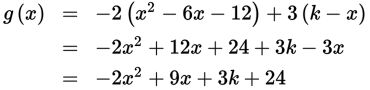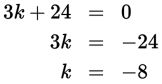# SAT Math Multiple Choice Question 902: Answer and Explanation

### Test Information

Question: 902

2. If g(x) = -2(x2 – 6x – 12) + 3(kx) and g(x) is evenly divisible by x, then what is the value of k?

• A. -8
• B. -3
• C. 2
• D. 3

Explanation:

A

Difficulty: Medium

Category: Passport to Advanced Math / Exponents

Strategic Advice: If a polynomial is evenly divisible by x, then x has to be a factor of the polynomial, which means that when simplified, every term must have an x in it. In other words, the polynomial cannot contain a constant term.

Getting to the Answer: Start by simplifying g(x). Be careful when distributing the -2:To ensure that there is no constant term, set 3k + 24 equal to 0 and solve for k.